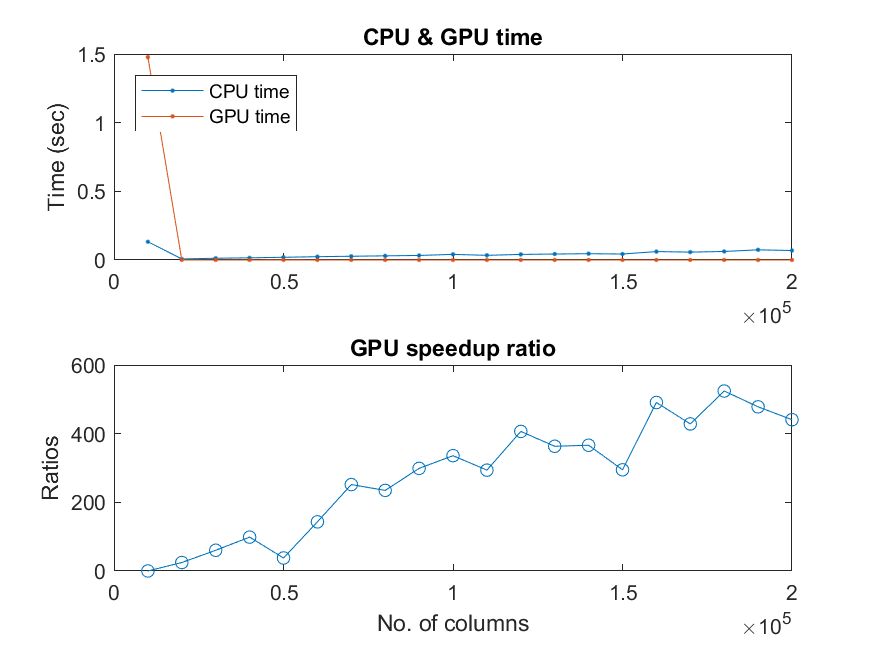## 2-3 嚙踝蕭嚙踝蕭嚙踝蕭GPU嚙踝蕭嚙踝蕭嚙踝蕭嚙踝蕭嚙踝蕭嚙踝蕭嚙踝蕭嚙?

GPU (graphic processing units) 的出現和普及，可說是近年來科學計算的最大變革，由於 GPU 具有大量平行處理的能力，所以對於某一些適合平行運算的應用，GPU 可說是最是適合不過了。

MATLAB 與 GPU 最相相關的兩個基本指令如下：內測試你的機器有幾張

• gpuDeviceCount: 可以回傳你的機器上面有幾張 GPU 卡（或顯卡）。
• gpuDevice: 可以回傳你的預設 GPU 卡的相關訊息。

Example 1: 02-程式碼與記憶體之最佳化/gpuDevice01.md = gpuDeviceCount g = gpuDevice d = 1 g = <a href="matlab:helpPopup parallel.gpu.CUDADevice" style="font-weight:bold">CUDADevice</a> with properties: Name: 'GeForce GTX 970M' Index: 1 ComputeCapability: '5.2' SupportsDouble: 1 DriverVersion: 7 ToolkitVersion: 6.5000 MaxThreadsPerBlock: 1024 MaxShmemPerBlock: 49152 MaxThreadBlockSize: [1024 1024 64] MaxGridSize: [2.1475e+09 65535 65535] SIMDWidth: 32 TotalMemory: 3.2212e+09 AvailableMemory: 2.9349e+09 MultiprocessorCount: 10 ClockRateKHz: 1038000 ComputeMode: 'Default' GPUOverlapsTransfers: 1 KernelExecutionTimeout: 1 CanMapHostMemory: 1 DeviceSupported: 1 DeviceSelected: 1

1. 使用 gpuArray 指令，將 MATLAB 工作空間的變數搬移到 GPU 的記憶體中。
2. 使用 GPU 記憶體中的變數來執行各種在 GPU 的運算。
3. 使用 gather 指令，將存放在 GPU 的變數搬移至 MATLAB 工作空間中。

Example 2: 02-程式碼與記憶體之最佳化/gpuStep01.ma=rand(100, 10000); b=rand(100, 10000)'; tic c=a*b; fprintf('CPU time = %g sec\n', toc); A=gpuArray(a); % Put a to GPU's memory B=gpuArray(b); % Put b to GPU's memory tic C=A*B; % Multiplication via GPU fprintf('GPU time = %g sec\n', toc); c2=gather(C); % Put C to MATLAB's workspace fprintf('isequal(c, c2) = %g\n', isequal(c, c2)); fprintf('Mean deviation = %g\n', mean(mean(abs(c-c2))));CPU time = 0.00463387 sec GPU time = 0.000350486 sec isequal(c, c2) = 0 Mean deviation = 5.55428e-13

1. GPU 的計算時間（不包含資料搬移的時間）大約只有 CPU 計算時間的 1/20。
2. GPU 計算結果和 CPU 不完全相同，但兩者的差異性極小。

Example 3: 02-程式碼與記憶體之最佳化/gpuSpeedup01.mfprintf('computer = %s\n', computer); fprintf('version = %s\n', version); % Speed test step=10000; colCounts=step*(1:1:20); for i=1:length(colCounts) fprintf('%d/%d\n', i, length(colCounts)); n=colCounts(i); a=rand(100, n); b=rand(100, n)'; myTic=tic; c=a*b; cpuTime(i)=toc(myTic); A=gpuArray(a); B=gpuArray(b); myTic=tic; C=A*B; gpuTime(i)=toc(myTic); end subplot(211); plot(colCounts, cpuTime, '.-', colCounts, gpuTime, '.-'); legend('CPU time', 'GPU time', 'location', 'northwest'); title('CPU & GPU time'); ylabel('Time (sec)'); subplot(212); plot(colCounts, cpuTime./gpuTime, 'o-'); title('GPU speedup ratio'); ylabel('Ratios'); xlabel('No. of columns');computer = PCWIN64 version = 9.3.0.651671 (R2017b) Prerelease 1/20 2/20 3/20 4/20 5/20 6/20 7/20 8/20 9/20 10/20 11/20 12/20 13/20 14/20 15/20 16/20 17/20 18/20 19/20 20/20Hint

（待續）

MATLAB程式設計：進階篇